Subscription# Directory

← Back

## SO Charts

A wrapper around the "echarts" Javascript library for creating charts.

### Rating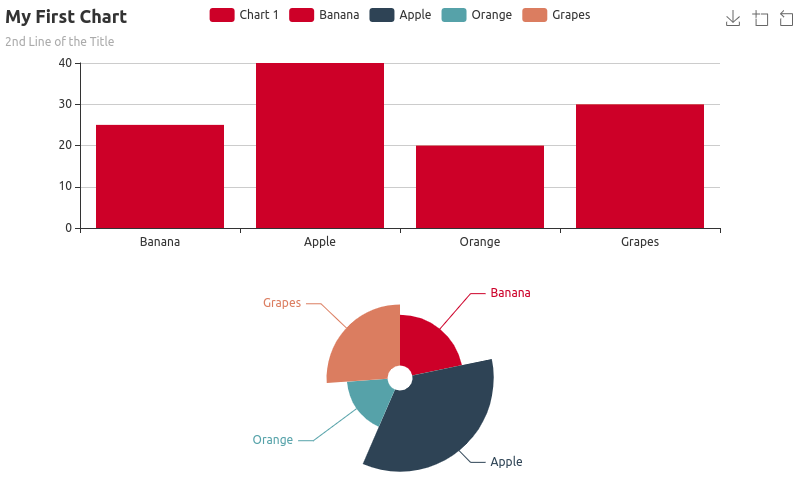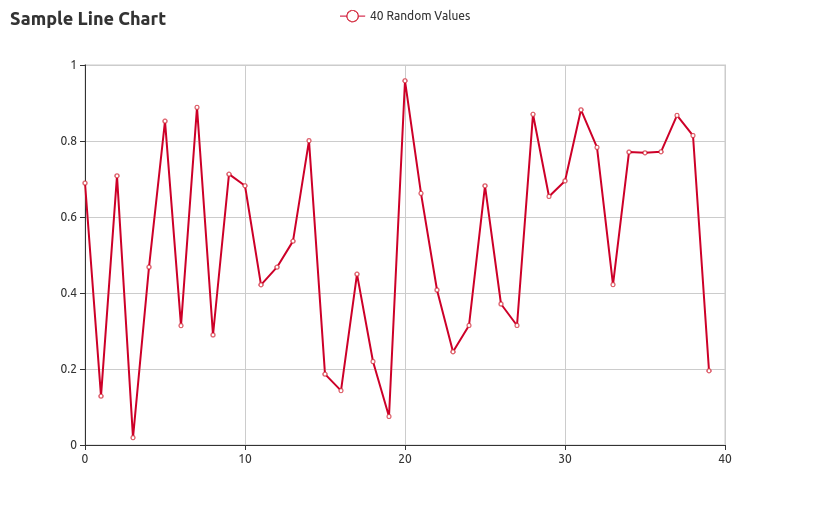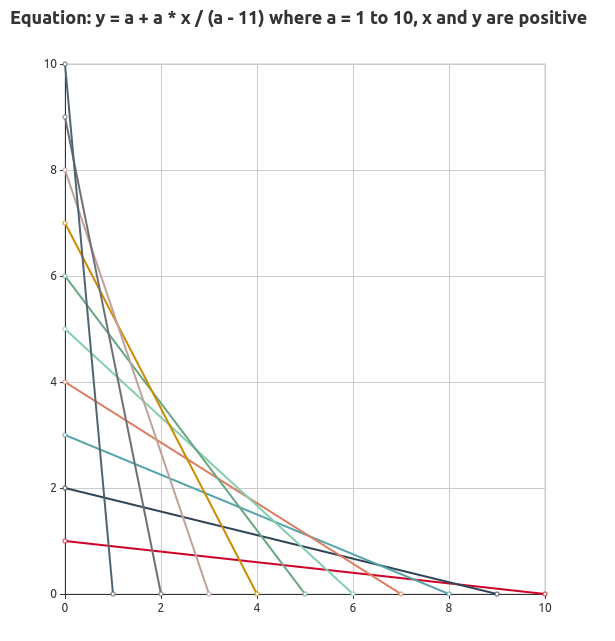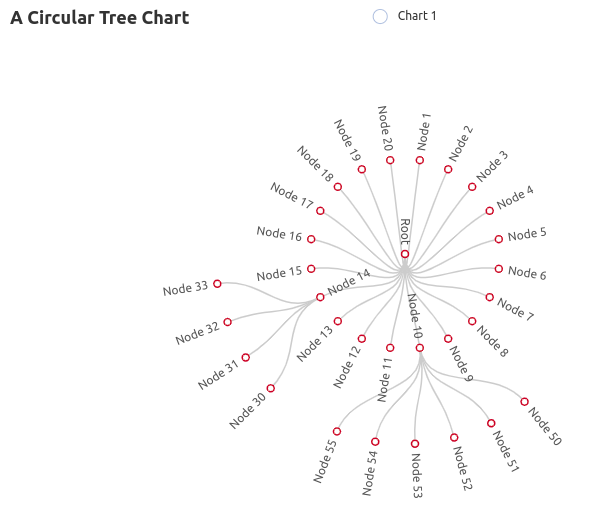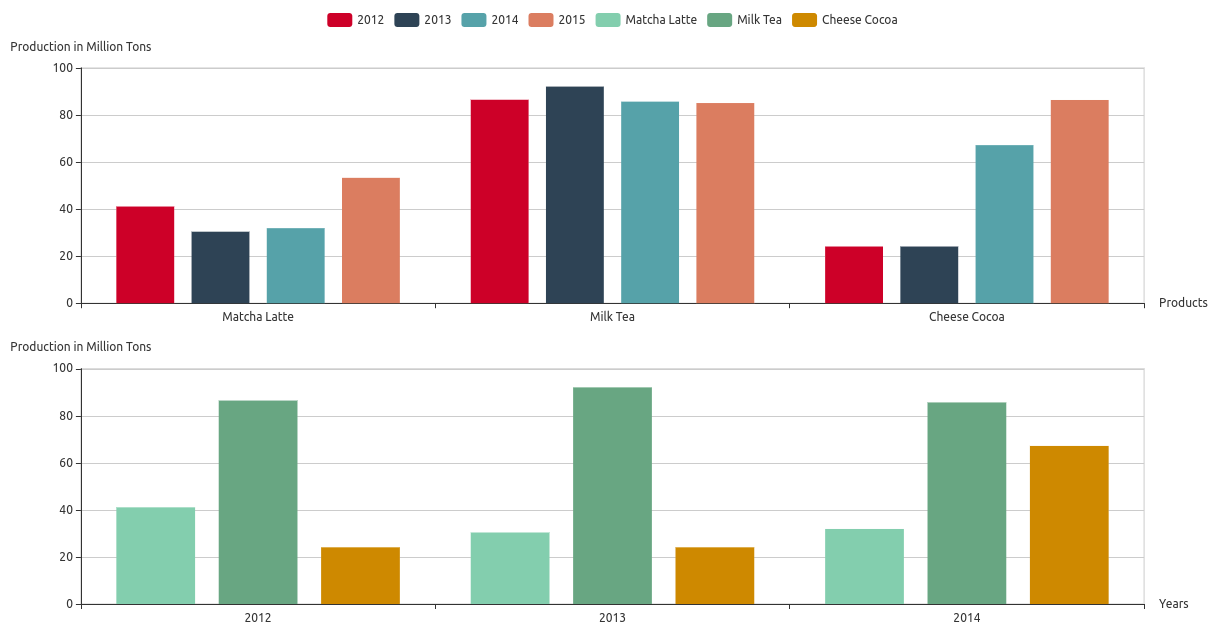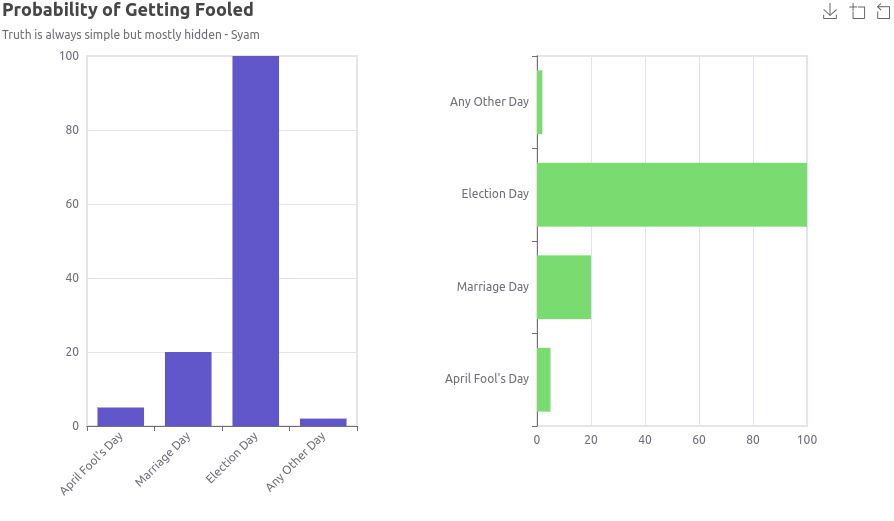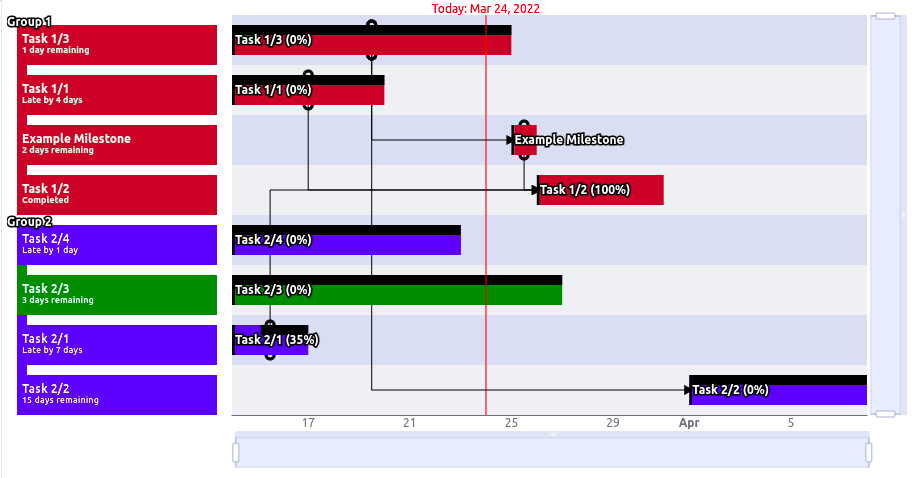# SO Charts

This add-on is a wrapper around the echarts Javascript library for using it with Vaadin Flow. The charts can be used just like any other Vaadin component.

"echarts" is quite a rich library and bringing all the available functionalities into this add-on will require quite some effort. However, my plan is to keep integrating more and more features into this add-on as and when I have some free time.

The client-side part of the add-on is a very thin LitElement wrapper around the "echarts" library. The SOChart class is the one that wraps that into the Java class to make it appear as a Vaadin Flow Component. Communication between them are through a couple of property change messages and a monolithic update call that carries all the chart data (including the configuration information) as a string parameter that can be JSONified. At the client-side, the string parameter received is used to create the structure for setting the options of the chart.

Just before sending the string containing the chart data and the configuration to the client-side, it is passed through a method - `customizeJSON(String)` - so that if someone wants to debug or customize it, it is very well possible. (Starting from 2.2.0, data can be transmitted separately and that can be intercepted via a new method - `customizeDataJSON(String)`).

Most classes in this add-on are either "chart components" or "chart component parts". All "chart components" can be added to the SOChart instance (the only Vaadin Flow Component). So, one instance of SOChart class (represents a "chart display") can be used for displaying any number of Charts (instances of Chart class - it is also a "chart component") and other "chart components".

Starting from version 2.1.0, various shapes, including texts and images, can be defined and added to the chart display.

Starting from version 2.2.0, a new method is added to transmit data separately whenever required.

Starting from version 2.3.0, a new method is added to transmit data separately for a specific chart whenever required.

Note: The online demo contains chart examples and other proprietary examples. You may use the keyword "chart" to filter out chart examples.

Notes to those who use Vaadin Spring Configuration: Please make sure that you update your `application.properties` if needed. It has been reported that this add-on may not work if the add-on package is not white-listed there. (Thanks to Marcus Merten for pointing this out). See here
Configuration example of application.properties (Thanks to Ryan Neuharth):

``````vaadin.whitelisted-packages = com.vaadin,org.vaadin,com.storedobject
``````

Credits to other contributors:
(1) Christian Asnel Ngoulla Sob
(2) Stefano Bossi
(3) Lazy Math Student

## Sample code

```// Creating a chart display area.
SOChart soChart = new SOChart();
soChart.setSize("800px", "500px");

// Let us define some inline data.
CategoryData labels = new CategoryData("Banana", "Apple", "Orange", "Grapes");
Data data = new Data(25, 40, 20, 30);

// We are going to create a couple of charts. So, each chart should be positioned
// appropriately.
// Create a self-positioning chart.
NightingaleRoseChart nc = new NightingaleRoseChart(labels, data);
Position p = new Position();
p.setTop(Size.percentage(50));
nc.setPosition(p); // Position it leaving 50% space at the top

BarChart bc = new BarChart(labels, data);
RectangularCoordinate rc;
rc  = new RectangularCoordinate(new XAxis(DataType.CATEGORY), new YAxis(DataType.NUMBER));
p = new Position();
p.setBottom(Size.percentage(55));
rc.setPosition(p); // Position it leaving 55% space at the bottom
bc.plotOn(rc); // Bar chart needs to be plotted on a coordinate system

// Just to demonstrate it, we are creating a "Download" and a "Zoom" toolbox button.
Toolbox toolbox = new Toolbox();

Title title = new Title("My First Chart");
title.setSubtext("2nd Line of the Title");

// Add the chart components to the chart display area.

```
```// Creating a chart display area
SOChart soChart = new SOChart();
soChart.setSize("800px", "500px");

// Generating some random values for a LineChart
Random random = new Random();
Data xValues = new Data(), yValues = new Data();
for(int x = 0; x < 40; x++) {
}
xValues.setName("X Values");
yValues.setName("Random Values");

// Line chart is initialized with the generated XY values
LineChart lineChart = new LineChart(xValues, yValues);
lineChart.setName("40 Random Values");

// Line chart needs a coordinate system to plot on
// We need Number-type for both X and Y axes in this case
XAxis xAxis = new XAxis(DataType.NUMBER);
YAxis yAxis = new YAxis(DataType.NUMBER);
RectangularCoordinate rc = new RectangularCoordinate(xAxis, yAxis);
lineChart.plotOn(rc);

// Add to the chart display area with a simple title

```
```// Creating a chart display area
SOChart soChart = new SOChart();
soChart.setSize("600px", "650px");

// Generating 10 set of values for 10 LineCharts for the equation:
// y = a + a * x / (a - 11) where a = 1 to 10, x and y are positive
LineChart[] lineCharts = new LineChart;
Data[] xValues = new Data[lineCharts.length];
Data[] yValues = new Data[lineCharts.length];
int i;
for(i = 0; i < lineCharts.length; i++) {
xValues[i] = new Data();
xValues[i].setName("X (a = " + (i + 1) + ")");
yValues[i] = new Data();
yValues[i].setName("Y (a = " + (i + 1) + ")");
}
// For each line chart, we need only 2 end-points (because they are straight lines).
int a;
for(i = 0; i < lineCharts.length; i++) {
a = i + 1;
}

// Line charts are initialized here
for(i = 0; i < lineCharts.length; i++) {
lineCharts[i] = new LineChart(xValues[i], yValues[i]);
lineCharts[i].setName("a = " + (i + 1));
}

// Line charts need a coordinate system to plot on
// We need Number-type for both X and Y axes in this case
XAxis xAxis = new XAxis(DataType.NUMBER);
YAxis yAxis = new YAxis(DataType.NUMBER);
RectangularCoordinate rc = new RectangularCoordinate(xAxis, yAxis);
for(i = 0; i < lineCharts.length; i++) {
lineCharts[i].plotOn(rc);
}

// Add a simple title too
soChart.add(new Title("Equation: y = a + a * x / (a - 11) where a = 1 to 10, x and y are positive"));

// We don't want any legends
soChart.disableDefaultLegend();

// Add it to my layout
```// Creating a chart display area
SOChart soChart = new SOChart();
soChart.setSize("800px", "500px");

// Tree chart
// (By default it assumes circular shape. Otherwise, we can set orientation)
// All values are randomly generated
TreeChart tc = new TreeChart();
TreeData td = new TreeData("Root", 1000);
tc.setTreeData(td);
Random r = new Random();
for(int i = 1; i < 21; i++) {
td.add(new TreeData("Node " + i, r.nextInt(500)));
}
TreeData td1 = td.get(13);
td = td.get(9);
for(int i = 50; i < 56; i++) {
td.add(new TreeData("Node " + i, r.nextInt(500)));
}
for(int i = 30; i < 34; i++) {
td1.add(new TreeData("Node " + i, r.nextInt(500)));
}

// Add to the chart display area with a simple title
soChart.add(tc, new Title("A Circular Tree Chart"));

// Finally, add it to my layout
```    // Creating a chart display area
SOChart soChart = new SOChart();
soChart.setSize("800px", "500px");

// To hold multiple charts
List<Chart> charts = new ArrayList<>();

// Create multiple charts
createCharts(charts);

// Add the chart component(s) to the chart display area

private void createCharts(List<Chart> charts) {
// Define a data matrix to hold production data.
DataMatrix dataMatrix = new DataMatrix("Production in Million Tons");
// Columns contain products
dataMatrix.setColumnNames("Matcha Latte", "Milk Tea", "Cheese Cocoa");
dataMatrix.setColumnDataName("Products");
// Rows contain years of production
dataMatrix.setRowNames("2012", "2013", "2014", "2015");
dataMatrix.setRowDataName("Years");

// Define axes
XAxis xAxisProduct = new XAxis(DataType.CATEGORY);
xAxisProduct.setName(dataMatrix.getColumnDataName());
XAxis xAxisYear = new XAxis(DataType.CATEGORY);
xAxisYear.setName(dataMatrix.getRowDataName());
YAxis yAxis = new YAxis(DataType.NUMBER);
yAxis.setName(dataMatrix.getName());

// First rectangular coordinate
RectangularCoordinate rc1 = new RectangularCoordinate();
rc1.getPosition(true)
.setBottom(Size.percentage(55)); // Position it leaving 55% space at the bottom
// Second rectangular coordinate
RectangularCoordinate rc2 = new RectangularCoordinate();
rc2.addAxis(xAxisYear, yAxis); // Same Y-axis is re-used here
rc2.getPosition(true).setTop(Size.percentage(55)); // Position it leaving 55% space at the top

// Bar chart variable
BarChart bc;

// Crate a bar chart for each data row
for (int i = 0; i < dataMatrix.getRowCount(); i++) {
bc = new BarChart(dataMatrix.getColumnNames(), dataMatrix.getRow(i));
bc.setName(dataMatrix.getRowName(i));
bc.plotOn(rc1);
}
// Crate a bar chart for each data column
for (int i = 0; i < dataMatrix.getColumnCount(); i++) {
bc = new BarChart(dataMatrix.getRowNames(), dataMatrix.getColumn(i));
bc.setName(dataMatrix.getColumnName(i));
bc.plotOn(rc2);
}
}
```
```import com.storedobject.chart.*;

@Route("")
public class ChartTest extends HorizontalLayout {

public ChartTest() {

// Creating a chart display area
SOChart soChart = new SOChart();
soChart.setSize("900px", "500px");

// Let us define some inline data
CategoryData labels =
new CategoryData("April Fool's Day", "Marriage Day", "Election Day", "Any Other Day");
Data data = new Data(5, 20, 100, 2);

// Axes
XAxis xAxis;
YAxis yAxis;

// Bar chart
BarChart bc1 = new BarChart(labels, data); // First bar chart
xAxis = new XAxis(labels);
xAxis.getLabel(true).setRotation(45);
yAxis = new YAxis(data);
RectangularCoordinate coordinate = new RectangularCoordinate(xAxis, yAxis);
bc1.plotOn(coordinate); // Bar chart needs to be plotted on a coordinate system
coordinate.getPosition(true).setRight(Size.percentage(60)); // Leave space on the right side

BarChart bc2 = new BarChart(data, labels); // Second bar chart
xAxis = new XAxis(data);
yAxis = new YAxis(labels);
coordinate = new RectangularCoordinate(xAxis, yAxis);
bc2.plotOn(coordinate); // Bar chart needs to be plotted on a coordinate system
coordinate.getPosition(true).setLeft(Size.percentage(60)); // Leave space on the left side

// Just to demonstrate it, we are creating a "Download" and a "Zoom" toolbox button
Toolbox toolbox = new Toolbox();

// Switching off the default legend
soChart.disableDefaultLegend();

Title title = new Title("Probability of Getting Fooled");
title.setSubtext("Truth is always simple but mostly hidden - Syam");

// Add the chart components to the chart display area

}
}
```
```// Examples of shapes that can be added to SOChart
ShapeGroup shapes = new ShapeGroup(); // Optional grouping
shapes.setZ(100);
shapes.getPosition(true).center();
Ring ring = new Ring(100, 20);
ring.setDraggable(true);
var style = ring.getStyle(true);
style.setStrokeColor(new Color("red"));
Text text = new Text("Hello World!\nHow are you?");
text.getStyle(true).setStrokeColor(new Color("red"));
Font font = new Font(Font.Family.fantasy(), Font.Size.x_large());
font.setStyle(Font.Style.OBLIQUE);
text.setFont(font);
Sector arc = new Sector(80, 0, 45);
arc.getStyle(true).setFillColor(new Color("yellow"));
Rectangle rectangle = new Rectangle(40, 30, 20);
rectangle.getStyle(true).setStrokeColor(new Color("red"));
BezierCurve bc = new BezierCurve(new Shape.Point(0, 0),
new Shape.Point(40, 40),
new Shape.Point(30, 20));
Polygon polygon = new Polygon(new Shape.Point(0, 0),
new Shape.Point(-20, -30),
new Shape.Point(10, -10));
polygon.useBezierSmoothening(0.6, true);

// Grouped together. Also, can be added individually.
shapes.add(ring, text, bc, rectangle, arc, polygon);

// Add to SOChart along with others like chart, legend, title etc.

### Version

Compiled with Java version 17 and Vaadin 23.2.15

Released
2023-06-17
Maturity
STABLE